# Functions

Here are some misc functions I've made that you can add to your own ghosts, if you like! Most of them are tools to help you make neat effects or to make coding easier, but some of them are just for fun. These functions were coded in YAYA with auto type convert turned on, so they may not work in AYA (or may need some adjusting).

# Holding Obsidian

Do you want YOUR GHOST to be able to pick up Dusty's cat, Obsidian? Great! Me too! Here's a video showing what it looks like, and a .zip download with instructions and examples. If you want to set up special commu dialogue between your ghost and Dusty, please let me know and I'd be glad to collaborate!

Demo video includes MiniDev by Zdzisiu.

# Interval Stacker

Do you use lots of intervals to create poses in your shell, and need an easier way to set up poses? Here's a .dic file and Saori that you can add to your ghost! Demonstrated with Zarla's Gaster because I haven't made any ghosts with lots of intervals. Uses a SERIKO parser made by Levidre.

# Simple Flags

If you have a lot of variables that only need to be set to 1 or 0, this set of functions can help you do that without needing lots of global variables! It also makes your flags easier to read.

```//Written by Zichqec https://zichqec.github.io/s-the-skeleton/index.html

//Usage: This is intended to take the place of single-use variables that only contain 0 or 1, used as flags for certain events and such. With these functions, you can store all those flags as a single array, meaning you can make as many flags as you want without having to really worry about save bloat. It can also make your code a bit more readable!

//You'll want to put 'MiscFlags = IARRAY' into your OnFirstBoot. That'll set up an empty array for flags to be stored in.
//When you want to check if a flag is set or not, you can do it like so:
//if Flag("My Flag") == 0
//or
//if Flag("My Flag") == 1

//Any flags that are NOT in the array are 0, and any flags that ARE in the array are 1. So, to set a flag to 1, you add it to the array like this:
//if Flag("My Flag") == 0; MiscFlags ,= "My Flag"

//You don't technically need the if check there, but it stops duplicate entries from being added. The ,= is also important; that adds a new element to an array. Don't use += for this!

//If you want to set a flag back to 0, you can remove it like so:
//RemoveFlag("My Flag")

//Flag names can be just about anything you want! They're just stored as strings. For example, for S I have "Saw Gaster with googly eyes", and for Lulo I have things like "Unlocked MP3 player" and "Unlocked journal". If you want to see what flags are set, you can just put %(MiscFlags) into script input, so it's helpful to have names that describe the flag!

Flag //Checks to see if a flag exists. Returns 1 if yes, returns 0 if no
{
if ASEARCH(_argv,MiscFlags) != -1; 1
else; 0
}

RemoveFlag //Removes all instances of a flag in the array.
{
_elements = ASEARCHEX(_argv,MiscFlags)
if ARRAYSIZE(_elements) > 0
{
for _i = ARRAYSIZE(_elements) - 1; _i >= 0; _i-- //Goes from the end of the list to the start, so the elements stay in the same positions while we erase
{
_current = _elements[_i]
MiscFlags[_current] = IARRAY
}
}
}

//Technical details:

//ASEARCH is a YAYA command that searches an array for the value you specify. If it doesn't find the value, it returns -1. If it finds the value, it returns the position in the array.

//ASEARCHEX is similar, but it finds all instances of the same value, and returns the positions as a new array.

//So, it IS possible to skip a step here and write if ASEARCH("My Flag",MiscFlags) != -1, to see if a flag is set. But != -1 is a bit confusing at times! So the Flag function here returns 1 if the flag is set, and 0 if it is not, to simplify things.

//With the RemoveFlag function, all it does is see how many instances of the value there are, then start erasing them from the end of the array working towards the start of the array, so that the array positions don't change as it erases. The reason I've done it this way is so that if someone accidentally adds the same flag to the array a bunch of times, all of them will be erased, so that the flag returns 0.
```

# OnRandomDressup

Have a lot of dressups, and want to offer your ghosts a way to pick a random set of dressups with a single click of a button? This set of functions can do that! Read the notes carefully, as this contains two other functions you may already have. (Currently does not work on AYA, maybe some day. If you have an AYA ghost and want to use this, let me know!)```//Written by Zichqec https://zichqec.github.io/s-the-skeleton/
//IMPORTANT NOTE: This also contains two functions you might already have - The built in SHIORI event OnNotifyDressupInfo, and my function ASUB. If you already have ASUB, just don't include this ASUB. They're the same. If you already have OnNotifyDressupInfo, you might have to modify it so that they don't conflict!

//This function will remove all the current dressups from a character, then pick random ones based on what categories of dressup are available. If the category is mustselect, it will always pick one. If it's multiple, it has a chance to pick multiple. And any categories that are not mustselect may be skipped over entirely, to increase variety.
//Note: You have to send it the number of the character you want to be dressed up as reference0! If you're just using this for a sakura, just always send it 0. 1 if you want the kero, 2 \p, etc.
OnRandomDressup
{
_command = ""
foreach currentdressups; _dressup //Gets the data for all current dressups and removes them
{
if _dressup[0,"|"] != TOSTR(reference0); continue
_category = _dressup[1,"|"]
_name = _dressup[2,"|"]
_command += "\![bind,%(_category),%(_name),0]"
}
"\p[%(reference0)]%(_command)\![done    removing]" //removes all current dressups - fake sakurascript tag here makes it easier to spot in the changelog, for debugging purposes
--
_currentcategories = IARRAY
foreach availabledressups; _dressup //For every dressup that the ghost has - This breaks up the big list of all the dressups into categories
{
if _dressup[0,"|"] != TOSTR(reference0); continue
_category = _dressup[1,"|"] //Gather the name and category of the current dressup
_name = _dressup[2,"|"]

_index = ASUB("%(_category)|",_currentcategories)
if _index == -1 //If the user is not in a submenu AND we haven't logged this category yet
{
if _dressup[3,"|"] == "mustselect" || _dressup[4,"|"] == "mustselect"; _adding += "!-!mustselect!-!" + "|" //Add the category to a list
if _dressup[3,"|"] == "multiple" || _dressup[4,"|"] == "multiple"; _adding += "!-!multiple!-!" + "|" //Add the category to a list

}
else
{
_currentcategories[_index] += "|" + _name
}

}
--
_command = ""
_skipchance = 3
foreach _currentcategories; _category //Picks a random dressup from every category
{
_temp = REPLACE(_category,"|",",") //Changes to comma delimiter
_temp = SPLIT(_temp,",") //Makes _temp into a general purpose array
_currentctg = _temp //Gets the name of the category

_loops = 1 //Always do the loop at least once, I suppose this is what a do-while loop is for haha
_multiple = 0
_mustselect = 0

if _temp == "!-!multiple!-!" || _temp == "!-!multiple!-!" //If it's a multiple option, mark it as such and up the number of loops to be 1 for every dressup available
{
_multicheck = 1
_loops = ARRAYSIZE(_temp) - 2
}
if _temp == "!-!mustselect!-!" || _temp == "!-!mustselect!-!" //If it's a mustselect, mark it as such. If it's also a multiple, reduce the number of times the loop is run, because this is taking up a slot of the array and we don't want to count it
{
_mustselect = 1
if _multicheck == 1; _loops--
}

if _temp == "!-!mustselect!-!" || _temp == "!-!multiple!-!" ; _temp = IARRAY //Erase these flags if they're present
if _temp == "!-!mustselect!-!" || _temp == "!-!multiple!-!" ; _temp = IARRAY
_temp = IARRAY //Erase the category name

for _i = 0; _i < _loops; _i++ //Run the loop as determined above
{
if RAND(_skipchance) != 0 //If it decides it should skip
{
if _i == 0 //If it's at the start of the category, skip the whole category
{
if _mustselect == 0; break
}
else //Otherwise, skip just this one
{
continue
}
}

_toadd = ANY(_temp) //If it doesn't skip, set _toadd to any dressup on the array
_num = LSO //LSO is a function that gets the element number ANY chose
_temp[_num] = IARRAY //Erase the dressup that was chosen

if _toadd == ""; continue //If it's empty, skip
}
}
"%(_command)"
}

OnNotifyDressupInfo
{
availabledressups = IARRAY //This one is to display the dressup menu
currentdressups = IARRAY //This one is so we can display which dressups are currently in use

foreach reference; _dressup //if you just write reference, you get all the references, apparently? Which is super useful
{
//Ok, so here's the deal. When it lists the dressups, the amount of options that can be specified _varies._ It can be empty, 1, 2, or 3. To the best of my knowledge. SO. This inner loop starts at 3 (because elements 0 1 and 2 are static), checks to see if the next element has a keyword, and skips it if it does. Then when it finds the on/off indicator, it breaks the loop. IT DIDNT HAVE TO BE THIS WAY.
_onoff = 0
_must = 0
_mult = 0
for _i = 3; _i < ARRAYSIZE(_dressup); _i++
{
if _dressup[_i] == "default" || _dressup[_i] == ""; continue
elseif _dressup[_i] == "mustselect" {_must = 1; continue}
elseif _dressup[_i] == "multiple" {_mult = 1; continue}
else; _onoff = _dressup[_i]; break
}
_toadd = _dressup + "|" + _dressup + "|" + _dressup
if _must == 1; _toadd += "|mustselect"
if _mult == 1; _toadd += "|multiple"
if _onoff == 1; currentdressups ,= _dressup + "|" + _dressup + "|" + _dressup
}
}

ASUB //ASEARCH but for substrings
{
_array = _argv //Takes in all the elements of the array
_array = IARRAY //erases _argv since that's the string we're searching for
for _i = 0; _i < ARRAYSIZE(_array); _i++
{
if _argv _in_ _array[_i]
{
_i
return
}
}
-1
}
```

# Parallel

Parallel is a function written to replace an old SAORI(?) that I couldn't get working. It lets multiple characters say different dialogues at the same time!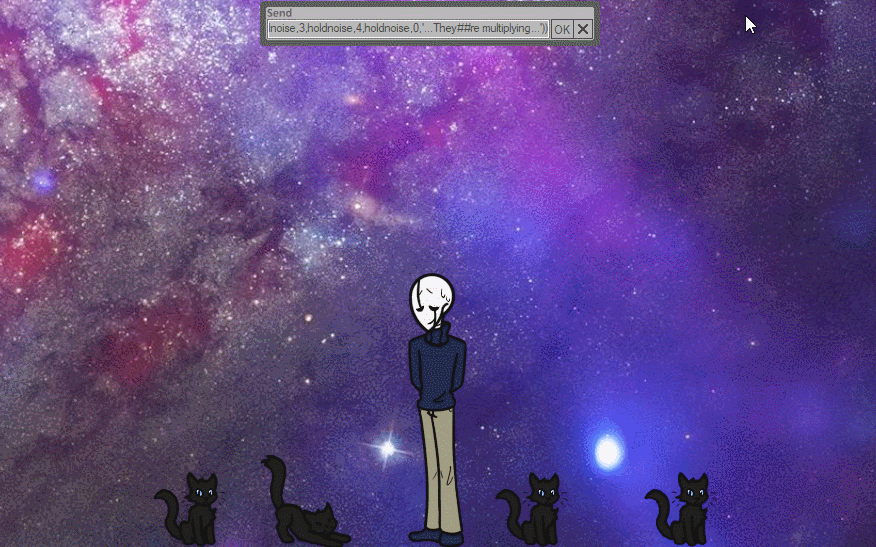```//Written by Zichqec https://zichqec.github.io/s-the-skeleton/

//This function is intended to replace the functionality of an old SAORI(?) that I found and couldn't get working. It lets multiple characters say different things at the same time.

//To call the function, you can either call the function in your code like this:
//Parallel(0,"Dialogue for the Sakura!",1,"Dialogue for the Kero!")
//Or in dialogue like this:
//"%(Parallel(0,'Dialogue for the Sakura!',1,'Dialogue for the Kero!'))"

//You can call any characters, in any order, and in any amount. Every other tag is a character tag, so you should write a number, the dialogue that goes with that number.
//%(Parallel(5,'Char 5 dialogue',37,'Char 37 dialogue',0,'Sakura dialogue',9,'Char 9 dialogue'))

//Please take care when writing the tags. I think I worked out all the infinite loop problems, but if your ghost ever crashes while using this function, do let me know and I'll see what I can do to fix it!

//You cannot write sakurascript tags within the Parallel tag, and if you want to write an apostrophe, you should write it as ##

Parallel
{
//Initializing variables
_output = ""
_chararray = IARRAY
_textarray = IARRAY

_i = 0
while _i < _argc //Sorts each argument into characters and dialogue
{
_chararray ,= _argv[_i]
_i++
_textarray ,= REPLACE(_argv[_i],"##","'") //Changes ## to an apostrophe, change it if you like
_i++
}
--
while ARRAYSIZE(_chararray) > 0 //Repeats until all dialogues have been outputted
{
_i = 0
_output += "\![quicksection,1]"
while _i < ARRAYSIZE(_chararray) //Loops through each char and displays a single character of dialogue, erases any dialogues/chars that have become empty
{
if STRLEN(_textarray[_i]) == 0
{
_chararray[_i] = IARRAY
_textarray[_i] = IARRAY
continue
}
_output += "\p[" + _chararray[_i] + "]" + SUBSTR(_textarray[_i],0,1)
_textarray[_i] = ERASE(_textarray[_i],0,1)
_i++
}
_output += "\![quicksection,0]\w1"
}
_output
}
```

# Wobble

Want to write weird, wobbly text? Here's a little function!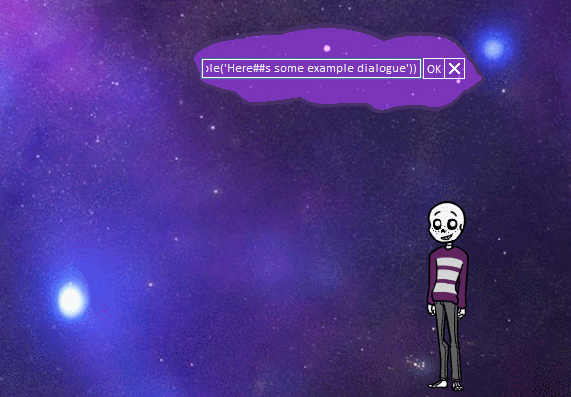```
//Written by Zichqec https://zichqec.github.io/s-the-skeleton/

//To call the function, you can either call the function in your code like this:
//Or in dialogue like this:

//You can also overwrite the default values by sending extra arguments after the dialogue. Argument 0 will always be the dialogue you want to run through the wobble function. Argument 1 is for random upper/lowercase (1 is on and 0 is off), arguments 2 and 3 are X and Y jitter respectively, argument 4 is the amount the font size can vary by per-letter, and argument 5 is if the font size can change up, down, or both (0 is both, 1 is up, and 2 is down).

//You may exclude any of these arguments, but you must include a blank argument where they would be.
//Example: Wobble("Spooooky","","10","30","2","1")
//This will skip the upper/lowercase argument.
//Arguments that are farthest to the right can be ignored entirely if you don't want them.
//Example: Wobble("Spooooky","1")
//This will add random upper/lowercase characters, but use default values for everything else. But if you want to add arguments for changing the font size and nothing else you'd have to write it like this:
//Example: Wobble("Spooooky","","","","2","1")

//If you want to write an apostrophe in dialogue, write ## and it'll be replaced with an apostrophe

Wobble
{
_argv = REPLACE(_argv,"##","'") //replaces ## with an apostrophe, you can change this if you want to use something else

//Default values - Set these to whatever you'd like, you can override them for each individual dialogue if you like
_UPlow = 0		//Controls if letters are randomly converted to upper/lowercase. 0 for off, 1 for on.
_Xjitter = 5	//Controls how much space can be between each letter. Higher numbers will be more spaced out.
_Yjitter = 5	//Controls how much letters can vary up and down. Higher numbers will be more spaced out.
_SizeJitter = 0 //Controls how much the font size can change per letter. Higher numbers will have more extreme variance.
_SizeUpDown = 1 //Controls if the font size can go up, down, or both. 0 for both, 1 for up, 2 for down.

if _argv != ""; _UPlow = _argv //These check if the user has sent special arguments, and if not, uses the default values
if _argv != ""; _Xjitter = _argv
if _argv != ""; _Yjitter = _argv
if _argv != ""; _SizeJitter = _argv
if _argv != ""; _SizeUpDown = _argv

_word = "" //Initialize display
for _i = 0; _i < STRLEN(_argv); _i++
{
_let = SUBSTR(_argv,_i,1) //Get the current letter
if TOINT(_UPlow) == 1 //If random upper/lower is on, flip a coin to determine which this letter will be
{
if RAND(2) == 1; _let = TOUPPER(_let); else; _let = TOLOWER(_let)
}
_X = RAND(_Xjitter) //Get random values for X, Y, and Size
_Y = RAND(_Yjitter)
_Sz = RAND(_SizeJitter)
if _SizeUpDown == 1 //Size only goes up
{
_Sz = "+" + _Sz
}
elseif _SizeUpDown == 2 //Size only goes down
{
_Sz = "-" + _Sz
}
else //Flip a coin on if the size should go up or down
{
if RAND(2) == 1; _Sz = "+" + _Sz; else; _Sz = "-" + _Sz
}
_word += "\_l[@%(_X),%(_Y)]\f[height,%(_Sz)]" + _let //Add to the display
}
_word
}
```

# Capitalize

This function will capitalize the first letter of whatever word/phrase you run through it. Most useful for envelopes when you need them to only be capitalized at the start of a sentence!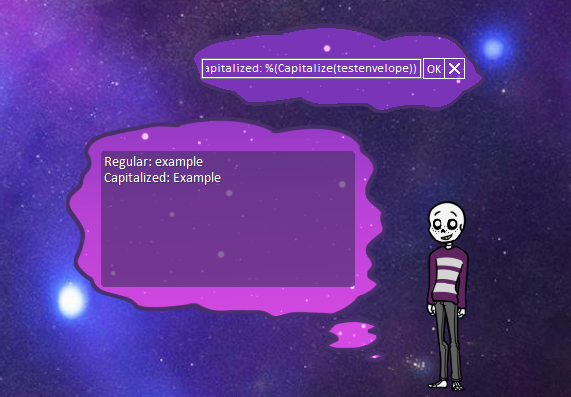```
//Written by Zichqec https://zichqec.github.io/s-the-skeleton/

//To call the function, you can either call the function in your code like this:
//Capitalize(SomeEnvelope) or Capitalize("some text")
//Or in dialogue like this:
//"%(Capitalize(SomeEnvelope))" or "%(Capitalize('some text'))"

Capitalize
{
_buffer = SUBSTR(_argv,0,1) //Storing the first character in _buffer
_argv = ERASE(_argv,0,1) //Erasing the first character from _argv
_argv = INSERT(_argv,0,TOUPPER(_buffer)) //Making the character in _buffer uppercase and inserting it back into _argv
_argv //Returns the capitalized word
}
```

# Fast

Want your ghost to speak some dialogue really quickly? Here's a simple function for that!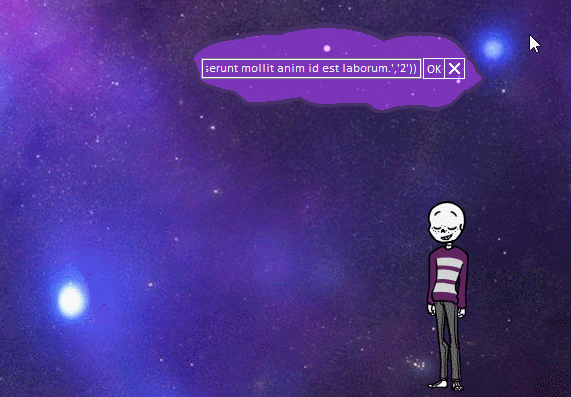```
//Written by Zichqec https://zichqec.github.io/s-the-skeleton/

//To call the function, you can either call the function in your code like this:
//Fast("some text",5)
//Or in dialogue like this:
//"%(Fast('some text',5))"
//Whatever you put as the second argument will be how many characters display at once. ONLY PUT NUMBERS AS THE SECOND ARGUMENT. I added some failsafes to be sure, but don't tempt fate.
//If you want to write an apostrophe in dialogue, write ## and it'll be replaced with an apostrophe

Fast
{
_argv = REPLACE(_argv,"##","'") //Replaces ## with an apostrophe, change it if you like
_output = ""
_argv = TOINT(_argv) //These two prevent infinite loops
if _argv < 1; _argv = 1
for _i = 0; _i < STRLEN(_argv); _i++
{
_output += "\![quicksection,true]"
_output += "%(SUBSTR(_argv,_i,_argv))"
_output += "\![quicksection,false]\w1"
_i += _argv - 1
}
_output
}
```

# Slow

Want your ghost to speak some dialogue really slowly without writing all those \w tags by hand? Here's a simple function for that!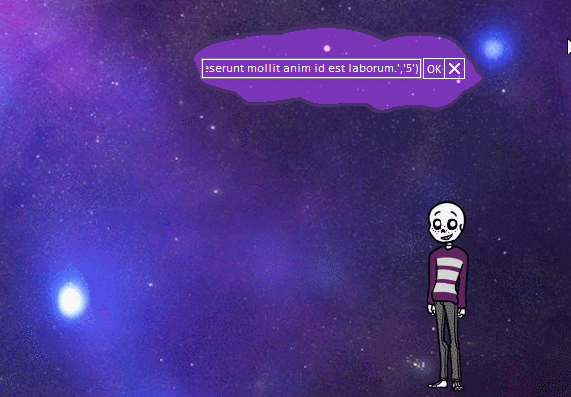```
//Written by Zichqec https://zichqec.github.io/s-the-skeleton/

//To call the function, you can either call the function in your code like this:
//Slow("some text",'5')
//Or in dialogue like this:
//"%(Slow('some text','5'))"

//If you want to use a precision \_w[] tag, which lets you write the ms directly, you can make the third argument be a 1. So, that'd look like this:
//"%(Slow('some text','50','1'))"
//Please note that simple \w tags are 50ms x the number you put. Precision tags are exactly the ms you specify.
//\w tags can only go up to 9, if you write anything higher they won't work properly.
//If you want to write an apostrophe in dialogue, write ## and it'll be replaced with an apostrophe

Slow
{
_argv = REPLACE(_argv,"##","'") //Replaces ## with an apostrophe, change it if you like
_output = ""
_p = ""
if _argv == "1" //If it's a precision \_w tag
{
_p = "\_w[" + _argv + "]"
}
else //Simple \w tag
{
_p = "\w" + _argv
}
for _i = 0; _i < STRLEN(_argv); _i++
{
_output += "%(SUBSTR(_argv,_i,1))%(_p)"
}
_output
}
```

# Mock

Want some dialogue displayed in mock case? Here's a little function for that!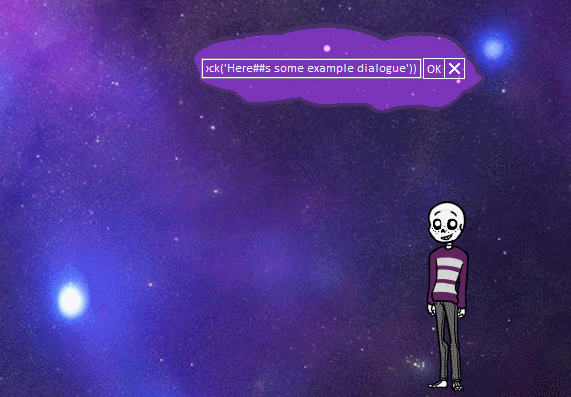```
//Written by Zichqec https://zichqec.github.io/s-the-skeleton/

//To call the function, you can either call the function in your code like this:
//Mock("some text")
//Or in dialogue like this:
//"%(Mock('some text'))"

//Note that by default, it will produce a 'perfect' mock case, meaning that it will change between lower and uppercase every character. If you want a 'random' mock case (which is really just randomcase, but some people say mock case is better with some chaos), put a 1 as the second argument. That'd look like this: "%(Mock('some text',1))"

//If you want to write an apostrophe in dialogue, write ## and it'll be replaced with an apostrophe

Mock
{
_argv = REPLACE(_argv,"##","'") //Replaces ## with an apostrophe, change it if you like
_output = ""
if TOINT(_argv) == 1 //Random mock case
{
for _i = 0; _i < STRLEN(_argv); _i++
{
_let = SUBSTR(_argv,_i,1)
if RAND(2) == 1
{
_output += TOLOWER(_let)
}
else
{
_output += TOUPPER(_let)
}
}
}
else //Perfect mock case
{
for _i = 0; _i < STRLEN(_argv); _i++
{
_let = SUBSTR(_argv,_i,1)
if _let == " "
{
_output += _let
continue
}
if _m % 2 == 0
{
_output += TOLOWER(_let)
}
else
{
_output += TOUPPER(_let)
}
_m++
}
}
_output
}
```

# Shuffle

Need to shuffle an array or a string? This function will do that automatically for you!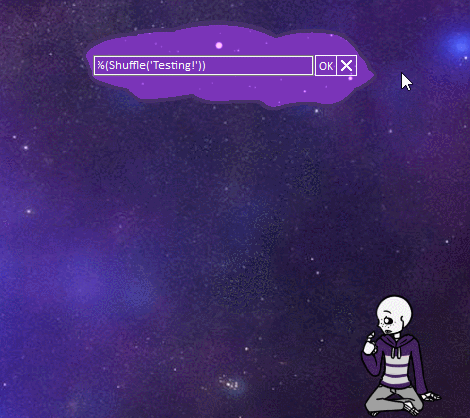```
//Written by Zichqec https://zichqec.github.io/s-the-skeleton/

//To call the function, you can either call the function in your code like this:
//Shuffle("A string!") or Shuffle(SomeArray)
//Or in dialogue like this:
//"%(Shuffle('A string!'))" or "%(Shuffle(SomeArray))"
//It will automatically detect strings vs arrays. General purpose arrays will have their elements shuffled. Anything else is treated as a string, including simple arrays, and will have the characters scrambled.
//If you send more than 1 argument, it will not work, so don't do that.

Shuffle
{
_output = IARRAY
if GETTYPE(_argv) == 4 //If it's an array. General purpose arrays only!
{
while ARRAYSIZE(_argv) > 0
{
_rand = RAND(ARRAYSIZE(_argv))
_output ,= _argv[_rand]
_argv[_rand] = IARRAY
}
}
else //If it's anything else it'll be treated as a string
{
_argv = TOSTR(_argv)
while STRLEN(_argv) > 0
{
_rand = RAND(STRLEN(_argv))
_output += SUBSTR(_argv,_rand,1)
_argv = ERASE(_argv,_rand,1)
}
}
_output
}
```

# CreepyText

Want to be able to write text in a really creepy and nonsensical way? Here's a simple function: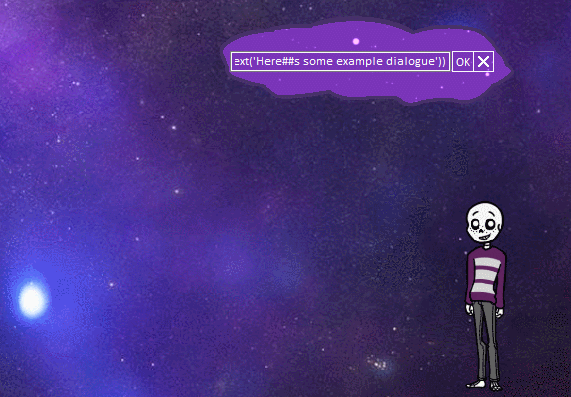```//Written by Zichqec https://zichqec.github.io/s-the-skeleton/

//To call the function, you can either call the function in your code like this:
//Or in dialogue like this:
//If you want to change the max \w tag value, put the number you want as the second argument. If you want to change the max x and y values, put them as the third and fourth argument, respectively. You can set defaults in the function below.

CreepyText
{
_argv = REPLACE(_argv,"##","'") //Replaces ## with an apostrophe, change it if you like
_display = ""
for _i = 0; _i < STRLEN(_argv); _i++
{
_w = 0
_x = 0
_y = 0
if _argv != ""; _w = RAND(_argv); else; _w = RAND(10)  //Set this to the maximum pause time you want, or comment it out if you don't want random pauses (Don't put it higher than 10 or you'll call invalid \w commands)
if _argv != ""; _x = RAND(_argv); else; _x = RAND(250) //Set this to the maximum X coordinate you want
if _argv != ""; _y = RAND(_argv); else; _y = RAND(100) //Set this to the maximum Y coordinate you want

_display += ("\_l[%(_x),%(_y)]" + SUBSTR(_argv,_i,1) + "\w%(_w)")
}
_display
}
```

# OnWander

I've set up a simple function that allows your ghost to move randomly around the monitor, while staying within the boundaries. See it here:

```//Written by Zichqec https://zichqec.github.io/s-the-skeleton/
//This function will make the ghost wander randomly on the current screen. If set properly, they should never go off either edge of the screen, even if it is not your primary monitor. Please note that I only have two monitors to test this with, it might break with 3 or more!
//I recommend making a hotkey for your test variable if you want to try this out, it's kinda fun tbh
//Please note; there is a bug where if you boot a ghost on a secondary monitor, they will move to the primary monitor if you ask them to wander without dragging them onto a different monitor. I can't think of a way to fix this, but if you think of something please tell me.
//To call this function, you can write %(OnWander('#')) in a line of dialogue, where # is the number of the ghost you want to move (0 for Sakura, 1 for Kero, 2 and up for extra chars). Alternatively, you can write OnWander("#") in code, the way you would write any other function.
//Dont forget to adjust the settings at the top of OnWanderCalc! You can set up individual settings for different characters by adding if checks for wanderChar, where 0 is Sakura, 1 is Kero, etc.
OnWander
{
wanderChar = TOINT(_argv)
if wanderLeft[wanderChar] == NULL; wanderLeft[wanderChar] = 0 //If somehow these are undefined, define them so the ghost doesn't go to the moon
if wanderRight[wanderChar] == NULL; wanderRight[wanderChar] = 500
"\![get,property,OnWanderCalc,currentghost.scope(%(_argv)).rect]"
}

OnWanderCalc
{
//Walk speed. The higher you make _walkRate, the slower the ghost will walk.
_walkRate = 200

//Ghost width. You need to adjust this so the ghost does not walk off the side of the screen. Set it to the width of your ghost's images. If your images are not the same width, this might behave strangely
_walkWidth = 230

//Minimum amount of pixels to move, so that the ghost doesn't move tiny, jarring amounts. Adjust as you like.
_walkMinimum = 200

//---Don't touch the rest of this, aside from the surfaces near the bottom!---
//{ If you're using notepad++, you can collapse this bracket so you don't have to see all that messy code

_XCoord = TOINT(reference0)
_char = wanderChar
_debug = "Started from %(_XCoord), left edge is %(wanderLeft[_char]), right edge is %(wanderRight[_char])\n"

_debug += "Char is %(_char)\n"
if nowscale != ""
{
_adjustedWidth = _walkWidth * (nowscale * 0.01)
}
else
{
}

_Left = TOINT(wanderLeft[_char])
_Right = TOINT(wanderRight[_char])
_Left -= wanderLeft[_char]
_Right -= wanderLeft[_char]
_debug += "wanderLeft[_char]: %(wanderLeft[_char])\n"
if _Right < 0
{
_Right *= -1
_debug += "_Right less than 0, inverted\n"
}
_RightRand = _Right
_RandX = RAND(_RightRand)
_RandX += TOINT(wanderLeft[_char])
_debug += "Moving to %(_RandX)\n"

_side = ""
if _RandX > _XCoord //If moving Right
{
_debug += "Moving right\n"
if _RandX - _XCoord < _walkMinimum //If it isn't moving at least the minimum distance to the right
{
_RandX = _XCoord + _walkMinimum
_debug += "Adjusted to the right\n"

if _RandX > (wanderRight[_char] - _adjustedWidth) //If it's past the right edge
{
_debug += "Stopped on the right edge\n"
}
}
}
elseif _RandX < _XCoord //If moving left
{
_debug += "Moving left\n"
_RandXN = _RandX
_XCoordN = _XCoord
if wanderLeft[_char] < 0
{
_RandXN = (_RandX * -1) //Inverting the negative numbers so we can math properly
_XCoordN = (_XCoord * -1)
_debug += "Inverted _XCoord and _RandX\n"
}

if _RandXN - _XCoordN < _walkMinimum //If it isn't moving at least the minimum distance to the left
{
_RandX = _XCoord - _walkMinimum
_debug += "Adjusted to the left, new destination %(_RandX)\n"

if _RandX <= TOINT(wanderLeft[_char]) //If it's past the left edge
{
_debug += "Stopped on the left edge\n"
_RandX = wanderLeft[_char]
}
}
}

if _RandX == _XCoord //If it fails to move at all
{
if _RandX == wanderLeft[_char] //If it's on the left edge
{
_debug += "Didn't move, stepped right\n"
_RandX += _walkMinimum
}
else //If it's on the right edge, or I guess if it happened to draw the exact same random coordinate as where it's standing
{
_RandX -= _walkMinimum
_debug += "Didn't move, stepped left\n"
}
}
_debug += "Stopped at %(_RandX)\n"

_walkTime = (_XCoord - _RandX) //Subtract the X we're moving to from the X we're starting at.
if _walkTime < 0; {_walkTime *= -1} //If this ends up being a negative number, make it into a positive one.
_walkTime /= 100 //Divide it by 100 so we're dealing with a small number.
_walkTime *= _walkRate //Multiply it by whatever the dev wants the walk rate to be.
if _walkTime == 0; {_walkTime = _walkRate} //If the walk time ends up being 0, make it at least the base _walkRate.

--
//}

//These ifs handle which direction the character is moving, and which char is moving. You can rearrange them if you like!
if _char == 0 //Moving the Sakura
{
if _RandX > _XCoord //Moving right
{
"\0\s" //Surface for walking to the right, adjust as needed.
}
else //Moving left
{
"\0\s" //Surface for walking to the left, adjust as needed.
}
}
elseif _char == 1 //Moving the Kero
{
if _RandX > _XCoord //Moving right
{
"\1\s" //Surface for walking to the right, adjust as needed.
}
else //Moving left
{
"\1\s" //Surface for walking to the left, adjust as needed.
}
}
else
{
"\p[%(_char)]" //Catch all for moving extra chars. If you want to move an extra char, you'll want to copy the if checks from the sakura/kero into here
}

--
"\![move,--X=%(_RandX),--time=%(_walkTime),--base=primaryscreen]" //This is the actual move command.
--
//This is whatever surface you want them to return to after they stop walking. If you want dialogue after they stop moving, it'll have to go here.
if _char == 0
{
"\0\s\e"
}
elseif _char == 1
{
"\1\s\e"
}
else
{
"\p[%(_char)]\s\e"
}
--
"\_q _RandX: %(_RandX),  _XCoord: %(_XCoord), Adjust: %(_adjust)  \n%(_debug)" //Debugging stuff that'll show up in the script log. You can comment it out or remove it, but the user will never see it anyways.
}

OnDisplayHandover //This sets the variables for if your ghost is moved between monitors. Gotta account for cases where people have different size monitors. I'd put this in etc.dic next to OnDisplayChange
{
wanderLeft[reference1] = reference3 //Tracks the left boundary of the current monitor for each char
wanderRight[reference1] = reference3 //Tracks the right boundary of the current monitor for each char
}

//Please also add this line to your OnShellScaling, so that it can properly adjust itself if the user changes the ghost's size. Don't forget to define it as 100 in OnFirstBoot, too!
nowscale = reference0
```